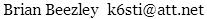# Q Meter Utilities

These Windows programs are for use with the HP 4342A Q meter, but they should work with other Q meters as well. Their main purpose is to compensate for the Q of an auxiliary capacitor that extends inductor measurement range. One program calculates the Q the meter would indicate if the auxiliary capacitor had no loss. Other programs calculate the capacitance and Q of small, large, or parallel auxiliary capacitors. Finally, a program calculates inductance from resonant frequency and capacitance. Use it to measure inductance at frequencies other than the spot frequencies where the inductance dial calibration is valid.

May 23, 202288–108 MHz